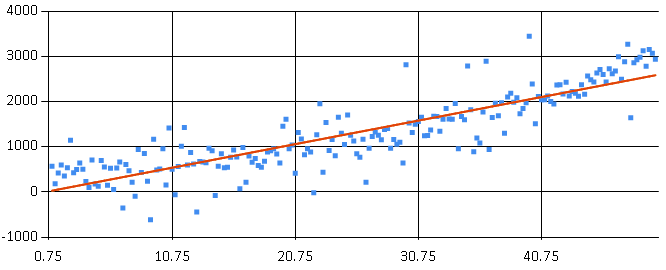# Linear Regression and F#

Today I look into performing linear regression using F#. The implementations of interest will be the MathNet and Accord.NET libraries. I assume you already know what linear regression is, but in can you need a refresher: Linear Regression. My goal is to provide a simple explanation of how to leverage some existing F# accessible libraries. Once you know some of the basic calling functions, you can go crazy with some of the other options these libraries have to offer.

Using Paket, here is a sample paket.dependencies file.

First I need to create some data. For this example, the formula is y = x^2 + noise + occasional outlier. The method is create 3 arrays representing x, noise, and outliers. It is a bit convoluted, but it allows me to show off a couple bits of functionality from F# and MathNet. The MathNet library includes methods to generate datasets, for the noise dataset `Generate.Normal` creates an array of numbers with a normal distribution. It is worth checking out the other diverse generation capabilities available. For outliers, I define an arbitrary 30% chance of a big spike in the data, as defined by pct and range variables. Then I use `|||>` and `Array.zip3` to combine the 3 element tuple of arrays into an array of 3 element tuples. Once in this format, a map is used to calculate the formula mentioned at the start.

Sidenote: If you’ve coded any F#, you know `|>`. But did you know there are other, similar operators: `||>` passes a tuple as two arguments, `|||>` passes a 3-tuple as 3 arguments.

### MathNet

Starting with the MathNet implementation, it is time for the regression fitting. The first option is `Fit.Line`, this takes the x and y data, fits a line and returns the associated intercept and slope that can be plugged into a y = mx + b formula. The second option is to use `Fit.LineFunc`. It also takes the x and y data to fit a line. The difference is it creates a delegate function that can be used to directly calculate.

Now it’s time to generate data based on the regression result. pData1 is calculated manually using the slope and intercept from the `Fit.Line` call. pData2 leverages the function delegate from `Fit.LineFunc`. I need to use `.Invoke` for performing the calculation.

The below code combines 3 charts; the original data, plus the regression lines. Some of the effect is lost, since the lines are ontop of each other, but you hopefully get the point.### Accord.NET

Now it is time to look at the Accord.NET implementation. Here,`OrdinaryLeastSquares` + `Learn` are used to determine line fitting. The result is a SimpleLinearRegression object.

Now it’s time to generate data based on the regression result. pData3 is calculated manually using the slope and intercept. pData4 is calculated directly by leveraging Accord’s `Transform()` function.

Again, the below code combines 3 charts; the original data, plus the regression lines. As expected, these graphs are identical.So, there it is. A couple ways to do linear regression, but there is more. What good is the regression model if you can’t perform scoring against the result. Luckily, MathNet and Accord.NET have several methods of comparing datasets. There are too many options to show them all here, but here are a couple examples scoring predicted data (pData1) versus actual data (yData). For reference: MathNet Distances and Accord.NET Distances. I recommend digging deeper to find the scoring method appropriate for your specific needs.

MathNet:

Accord.NET:

I hope this has been helpful if you’re venturing into F# and regression.